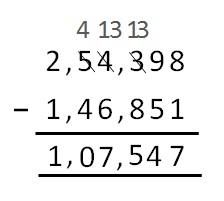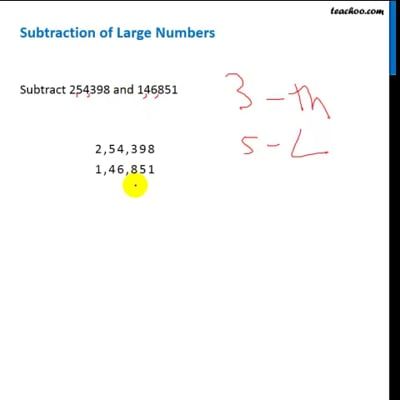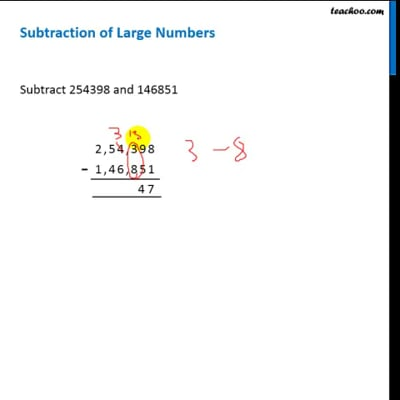Subtraction of large numbers

Chapter 1 Class 6 Knowing our Numbers
Concept wise

## Subtraction of Large Numbers

Subtract 254398 and 146851So, subtraction is 1, 07, 547This video is only available for Teachoo black usersThis video is only available for Teachoo black users

Solve all your doubts with Teachoo Black (new monthly pack available now!)

### Transcript

Subtraction of Large Numbers Suppose we want to subtract 254398 and 146851 We follow these steps 1. We write the numbers on top of each other (one digit below the other) 2. We start from the right, go towards the left 3. First we subtract 8 & 1, we get 7. We write 7 below 8 and 1 4. Then, we subtract 9 & 5, we get 4. We write 4 below 9 and 5 5. Now, we will subtract 3 and 8, but 3 is less than 8. So, it will need help from the next number on the left, 4 will become 3. And this 3 will become 13. So, we subtract 13 and 8. We get 5, so we write 5 below the digits 6. Now, we will subtract 3 and 6, but 3 is less than 6. So, it will need help from the next number on the left, 5 will become 4. And this 3 will become 13. So, we subtract 13 and 6. We get 7, so we write 7 below the digits 6. Now, we will subtract 4 and 4, we get 0. so we write 0 below the digits 7. We subtract 2 and 1, we get 1. We write 1 below So, subtraction is 1, 07, 547